# 【第一股票公式】请问昨天为该股的第一个涨停板选股公式怎么写？

BU:=CROSS(买线,卖线);
SEL:=CROSS(卖线,买线);
DRAWICON(BU,L-0.1,1);
DRAWICON(SEL,H+0.1,2);
STICKLINE(买线>=卖线,LOW,HIGH,0.0,1),COLORRED;
STICKLINE(买线>=卖线,CLOSE,OPEN,2,1),COLORRED;
STICKLINE(买线<卖线,LOW,HIGH,0.0,1),COLORGREEN;
STICKLINE(买线<卖线,CLOSE,OPEN,2,1),COLORGREEN;

LC:=REF(CLOSE,1);
RSI:=((SMA(MAX((CLOSE - LC),0),3,1) / SMA(ABS((CLOSE - LC)),3,1))*100);
FF:=EMA(CLOSE,3);
MA15:=EMA(CLOSE,21);

H1:=高1-(高1-低1)*0.191;
H2:=高1-(高1-低1)*0.382;
H3:=高1-(高1-低1)*0.5;
H4:=高1-(高1-低1)*0.618;
H5:=高1-(高1-低1)*0.809;

VAR1:=SMA(AMOUNT,10,1)/1000000;

HHJSJDA:=(3*CLOSE+OPEN+LOW+HIGH)/6;
HHJSJDB:=(20*HHJSJDA+19*REF(HHJSJDA,1)+18*REF(HHJSJDA,2)+17*REF(HHJSJDA,3)+16*REF(HHJSJDA,4)+15*REF(HHJSJDA,5)+14*REF(HHJSJDA,6)
+13*REF(HHJSJDA,7)+12*REF(HHJSJDA,8)+11*REF(HHJSJDA,9)+10*REF(HHJSJDA,10)+9*REF(HHJSJDA,11)+8*REF(HHJSJDA,12)
+7*REF(HHJSJDA,13)+6*REF(HHJSJDA,14)+5*REF(HHJSJDA,15)+4*REF(HHJSJDA,16)+3*REF(HHJSJDA,17)+2*REF(HHJSJDA,18)+
REF(HHJSJDA,20))/210,NODRAW;
N:=35;M:=35;N1:=3;
B1:=(HHV(H,N)-C)/(HHV(H,N)-LLV(LOW,N))*100- M;
B2:=SMA(B1,N,1)+100;
B3:=(C-LLV(L,N))/(HHV(H,N)- LLV(L,N))*100;
B4:=SMA(B3,3,1);
B5:=SMA(B4,3,1)+100;
B6:=B5-B2;

{第3个副图}

MMS:=MA(SMA((L+H+2*C)/4-REF((L+H+2*C)/4,1),5,1)/SMA(ABS((L+H+2*C)/4-REF((L+H+2*C)/4,1)),5,1)*100,1)+50;

G1:=IF(-MMS>95,0,20);

UOO:=(-(((CLOSE-LLV(CLOSE,7))/(HHV(CLOSE,7)-LLV(CLOSE,7))*100=100)>0 AND G1)+1);

KS:=(((CLOSE - EMA(CLOSE,13)) / EMA(CLOSE,13)) * (0 - 100));

KS1:=IF(BARSCOUNT(C)=1,C/10000,(1-C/SMA(C,13,1))*100);

AA:=-100*(趋势线-CLOSE)/趋势线;

AC:=(EMA(AA,5) );

SSL:=EMA(CLOSE,3);

MMV:=EMA(((SLOPE(CLOSE,22) * 20) + CLOSE),55);

MM:=MA(MMV,1);

SA:=(MM*0.985);

SS:=(MA(CLOSE,5));

DD:=(MA(CLOSE,13));

GG:=(MA(CLOSE,21));

JJ:=(上开+上高+上低)/3;

A:=EMA(JJ,4);

B:=REF(A,1);

VA1:=EMA(上收,8);

VA2:=EMA(上收,20);

DRAWTEXT(CROSS(ZIG(3,8),REF(ZIG(3,8),1)),LOW*0.978,'★绝佳') ,LINETHICK2,COLORRED;

DRAWTEXT(CROSS(REF(ZIG(3,10),1),ZIG(3,10)),HIGH,'★卖吧'),COLORGREEN;

DRAWTEXT(CROSS(VA1,VA2)AND(C>=O)AND 黄金线>REF(黄金线,1),VA1*0.97,'★买'),COLORFFFF00;

T1:=MA(上收,20)>=REF(MA(上收,20),1);

T2:=CROSS(选手A,箱底A) AND 箱顶A/箱底A>100/100 AND T1;

T3:=CROSS(箱顶A,选手A) AND 箱顶A/箱底A>100/100;

T4:=CROSS(选手,箱底) AND 箱顶/箱底>110/100;

T5:=CROSS(箱顶,选手) AND 箱顶/箱底>110/100;

VAR1:=(上收-LLV(上低,27))/(HHV(上高,27)-LLV(上低,27))*100;

VAR2:=SMA(VAR1,3,1);

VAR3:=SMA(VAR2,3,1);

VAR4:=SMA(VAR3,3,1);

DRAWTEXT(CROSS(VAR3,VAR4)AND(C>=O)AND VAR3<40 AND UOO>0,LOW*0.96,''),COLORF00FF0;

STICKLINE(C>=O,C,O,2.5,0),COLORRED;

STICKLINE(C>=O,H,L,0,0),COLORYELLOW;

STICKLINE(C<=O,C,O,2.5,0),COLORGREEN;

STICKLINE(C<=O,H,L,0,0),COLORFFFF00;

DRAWTEXT(CROSS(买线,卖线)AND 箱顶>=REF(箱顶,1),LOW*0.95,'★跟吧'),COLOR00FFFF;

STICKLINE(CROSS(买线,卖线),OPEN,CLOSE,3,0),COLOR00FFFF;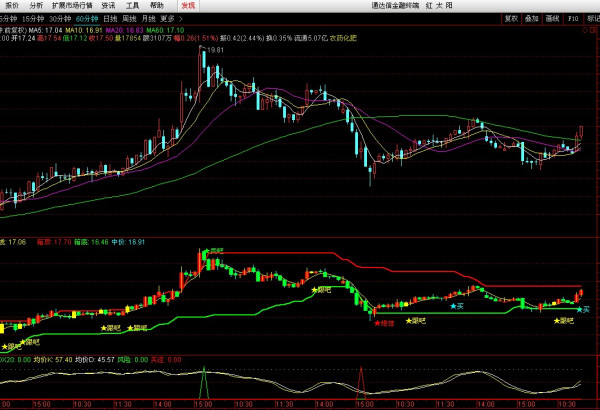`能加我交流吗2496791991 你这个公式我带进去显示错误`

`通达信软件适合使用`

`不行啊 我带进去 错误`

（一）现金流贴现模型

1、一般公式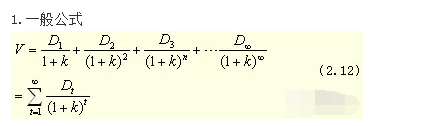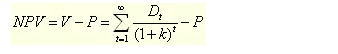2、内部收益（二）零增长模型

1、公式　假定每年支付的股利相同，股利增长率等于零，即g=0。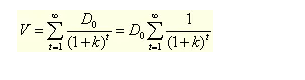2、内部收益率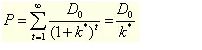3、应用

（三）不变增长模型

1、公式2、内部收益率（K*）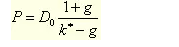3、应用

（四）可变增长模型

1、二元增长模型假定在时间 L以前，股息以一个不变的增长速度g1增长；在时间L后，股息以另一个不变的增长速度g2增长。在此假定下，我们可以建立二元可变增长模型：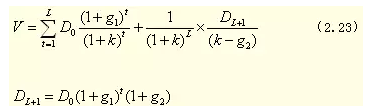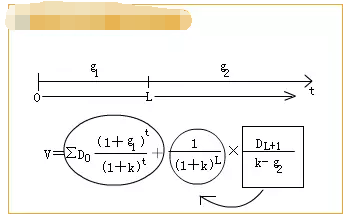2、内部收益率

3、应用if(条件and涨幅大于等于5%)